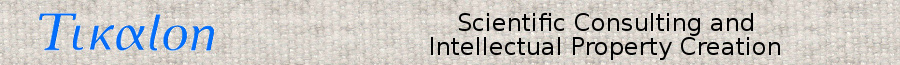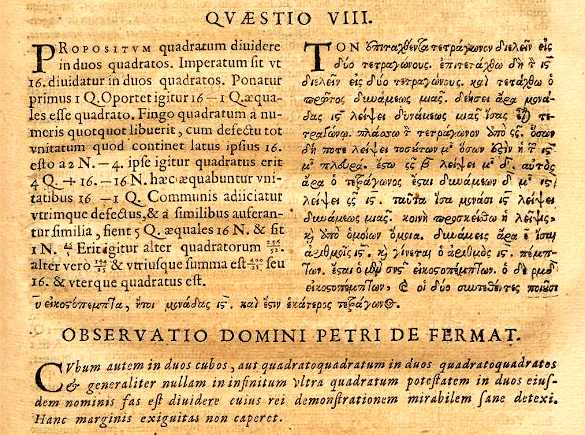### Sum of Three Cubes

March 14, 2022

Most number theory conjectures are easy to understand, but they are often very difficult to prove. One example of this is Fermat's Last Theorem, a conjecture that there are no three positive integers, a, b, and c, that satisfy the equation
an + bn = cn
for any integer n > 2. This conjecture is named after mathematician, Pierre de Fermat (1607-1665), who claimed to have an unpublished proof around 1637. In retrospect, this claim is quite unlikely. The actual proof, in 1995 by Andrew Wiles, is 129 pages long.

There are a few number theory conjectures that are both simple to state and simple to prove, one example of which is the conjecture that the square of any odd number is also odd. Anecdotal evidence from some odd numbers such as 3 (32 = 9), 11 (112 = 121), and 314159 (3141592 = 98695877281) is apparent, but that's not a proof. The actual proof is to create an odd number by adding one to an even number, n = 2k + 1. Then
n2 = 4k2 + 4k + 1

n2 = 2(2k2 + 2k) + 1
so, n2 is odd, since it's the sum of an even number plus one.

Diophantine equations are equations in which integer solutions are required. These equations as named after the 3rd century mathematician, Diophantus of Alexandria (c.284-c.298), who studied such equations and compiled them in his book, Arithmetica. Unlike later mathematics books, Arithmetica was a compilation of problems with singular solutions instead of a tutorial on solving such problems in general. Fermat had a copy of Arithmetica, and this is where he wrote his declaration that he had solved his Last Theorem conjecture (see figure)Page 61 of Arithmetica by Diophantus. Fermat's "Last Theorem" note, as published in this edition, appeared after Problem VIII of Book II. Fermat's note reads in translation, "It is impossible to separate a cube into two cubes, or a fourth power into two fourth powers, or in general, any power higher than the second, into two like powers. I have discovered a truly marvelous proof of this, which this margin is too narrow to contain." (Portion of a Wikimedia Commons image. Click for larger image.)

Problem VIII of Book II in Arithmetica is interesting in its own right, since it explains how to divide a square into a sum of two squares. In the question, Diophantus takes the square to be 16. If the first of the summed squares is x2, then the second would be 16 - x2. Diophantus then goes through a complicated method to find that x = 16/5 (view this Wikipedia page for details), with the result that one square is 256/25 and the other 144/25.

Diophantus also appears to have known that every number can be written as the sum of the squares of four integers, the proof of which is the 1770 Lagrange's four-square theorem by Joseph-Louis Lagrange(1736-1813). This leads us to the conjecture involving a Diophantine equation that any number can be written as the sum of three cubes. The Diophantine equation is written k = x3 + y3 + z3, in which k is the target number This idea seems strange at first until we remember that the cube of a negative number is also a negative number, so we can have very large numbers giving us small numbers in the summation.

A start in computer-assisted search for solutions to the three cubes conjecture was a 1955 effort for 0 ≤ k ≤ 100 and x, y, z absolute magnitudes below 3164 by Miller and Woollett at the Cambridge University Mathematical Laboratory using an EDSAC computer in which they found 345 solutions. A multiplication on the EDSAC took 6 msec. A 2016 study by Sander G. Huisman using about 100,000 hours of computer time on a variety of machines produced the first solution for z = 74, which left just z = 33 and z = 43 as the last numbers up through 100 that were unsolved. While 100 can be expressed quite simply with x = -6, y = -3. and z = 7, the following table lists some of the larger number solutions for k through 100. Reference 2 has a list of solutions for most values of k up to 999.

Sum of Three Cubes Conjecture
k x y z
7 -168218632243945 -61605392145082 170928876351450
9 -579503130257826 47158155506281 579399014699414
20 -156759508867952 90524105730987 145971698874625
35 -244965503743381 82852212729479 241764631510633
46 -1042925392876187 -2525776940964 1042925397814257
55 -928487831742068 -272851005732953 936276531557204
63 -545743190147561 -287677064051260 571183926715614
70 -1381417714821543 -223954055560540 1383376964067653
74 -284650292555885 66229832190556 283450105697727
90 -455329473688079 225963644244842 435967889130081
97 -478725341478772 -438717423067543 579049714409178
99 -740582589889197 -123796510872653 741733872643349
100 -588182578216077 -183057438103967 594034558164966

A solution for k = 33 was discovered in March, 2019, by British mathematician. Andrew R. Booker (b. 1976), who is professor of pure mathematics at the University of Bristol (Bristol, UK). Booker found that 33 can be expressed as the sum of cubes of 8 866 128 975 287 528, −8 778 405 442 862 239, and −2 736 111 468 807 040, and that left 42 as the last k through 100 that was unsolved.[4-5] Booker achieved this result by creating an algorithm that searched about twenty times faster than brute force. Science fiction fans will recognize that 42 is the Answer to the Ultimate Question of Life, the Universe, and Everything, as found in the The Hitchhiker's Guide to the Galaxy by Douglas Adams (1952-2001).

The solution for k = 42 was not long in coming, since a collaboration between Andrew Sutherland, a principal research scientist in the Department of Mathematics at MIT, and Andrew Booker discovered just a few months later that 42 can be expressed as the sum of cubes of -80 538 738 812 075 974, 80 435 758 145 817 515, and 12 602 123 297 335 631. Their computer power was a worldwide set of more than 400,000 personal computers of volunteers organized by the UK-based Charity Engine. There are now just ten numbers through 1000 that are unsolved, the next being 114.

### References:

Linked Keywords: Number theory; conjecture; mathematical proof; prove; Fermat's Last Theorem; natural number; positive integer; equation; mathematician; Pierre de Fermat (1607-1665); academic publishing; unpublished<; Andrew Wiles; square (algebra); parity (mathematics); odd number; anecdotal evidence; even number; Diophantine equation; integer; 3rd century; Diophantus of Alexandria (c.284-c.298); book; Arithmetica; mathematics; tutorial; Page 61 of Arithmetica by Diophantus containing Fermat's 'Last Theorem' note; edition; Diophantus II.VIII; Problem VIII of Book II; translation; cube (algebra); exponentiation; power; margin (typography); Wikimedia Commons; division (mathematics); divide; addition; sum; Lagrange's four-square theorem; Joseph-Louis Lagrange(1736-1813); computer-assisted; magnitude (mathematics); absolute magnitude; Cambridge University Mathematical Laboratory; EDSAC computer; multiplication; millisecond; msec; Sander G. Huisman; Great Britain; British; Andrew R. Booker (b. 1976); professor; pure mathematics; University of Bristol (Bristol, UK); algorithm; brute-force search; brute force; science fiction; fan (person); Answer to the Ultimate Question of Life, the Universe, and Everything; The Hitchhiker's Guide to the Galaxy; Douglas Adams (1952-2001); collaboration; Andrew Sutherland; research scientist; Department of Mathematics at MIT; personal computers; volunteer; United Kingdom; UK-based; Charity Engine; J. C. P. Miller and M. F. C. Woollett, "Solutions of the Diophantine Equation: x3+y3+z3=k," J. London Mathematical Society, vol. 1-30, no. 1 (January, 1955), pp. 101-110, https://doi.org/10.1112/jlms/s1-30.1.101.